# Exploring Root Solutions

Roots of numbers are weird. They never really made sense to me until I attempted to dig deep into the subject. It's a lot more complicated than on the surface.

Let's start with the square root. When you are looking for the square root of a number, you are asking a question: "What value, multiplied by itself, will give me that starting number?" Given some output, find a possible input. Remember that multiple outputs for a single input isn't sensible, but multiple inputs can have the same output. The number of solutions for finding even a square root of a number can be anywhere from 0 to infinite, depending on what you allow.

How about the square root of 1? 1 is a unit, so multiplying it by itself always gives you back 1. 1 is an easy solution to this. But there is another solution, which you may know already. Let me demonstrate below.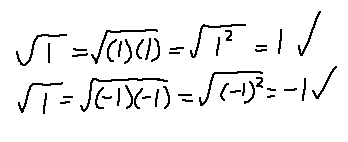By splitting 1 into -1*-1 under the root, that shows that -1 is also a valid solution. Even though I started with the same structure, I ended up with two different answers depending on how I represented 1! I'll come back to this later.

The square root of -1 is famously known to be i, the imaginary number. How does this make sense? Well, it doesn't, if you're restricted to using only real numbers. i is a value that is outside of the real numbers, which can be represented on a number line. The imaginary numbers is completely separate from the number line, and can therefore be represented as a line perpendicular to the reals. The combination of the reals and the imaginaries gives us the complex plane. I recommend reading more about this complex plane and multiplying complex numbers before continuing if you aren't familiar with it.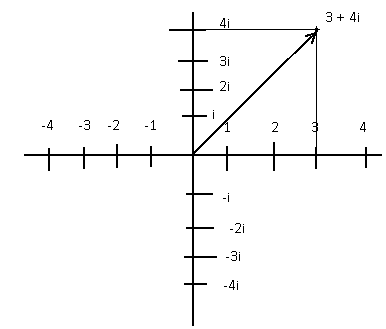Basically, the complex plane lets us play with numbers as if they were 2 dimensional vectors. When messing with complex numbers in particular, performing multiplication on them causes not only an increase in the absolute value of that vector (the distance from the origin point), but also a rotation in that vector.

Let's play with only unit complex numbers, so that their magnitude is 1 so that the magnitude will not change on multiplications. Multiplying two unit complex numbers together causes the result to have an angle equal to the sum of the two numbers. Here's an example: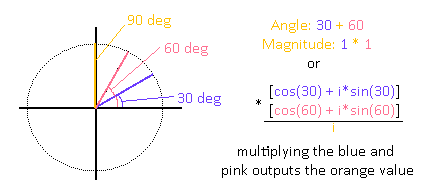I represented the result in two ways. We will be using the Angle/Magnitude representation of complex numbers, as they will be much more intuitive for us. One thing to note is that you need to handle complex numbers in a special way when it comes to multiplication if you're going to represent them as vectors, since there's more than one way you can multiply two vectors together. Anyways, this framework will help us finally understand the square root of -1.

Two values need to be multiplied together to equate to -1. Now that we have the complex plane, we can extend out into that area to help us find more solutions! "i" simply means having a magnitude of 1 and an Angle of 90 degrees in this case. What happens if you multiply i by itself?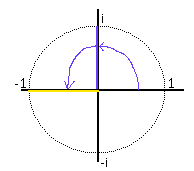The result of multiplying two "i"s is the same as adding together two quarter rotations around the unit circle to get a half rotation! This half rotation happens to land us on the real value -1. This quarter rotation, represented as "i", is therefore a solution to the square root of -1. There is actually another solution, since you can simply take two rotations clockwise instead of counter-clockwise, the result of which also leads to -1. That half-rotation in the other direction represents the value "-i". So, the square root of -1 actually has two solutions, both positive and negative "i".

So, back to the square root of 1. If we use the complex plane, then we can also find multiple solutions. -1 was one of them, which is basically like taking two half-rotations around the unit circle to reach back to 1. You could also take two full rotations around the circle twice to get you back at 1. Or take three full rotations twice, etc. In our magnitude/angle representation of all the solutions we can find, they would be: Magnitude 1 and Angle 180 deg, Magnitude 1 and Angle 360 deg, Magnitude 1 and Angle 720 deg, Magnitude 1 and Angle 1080 deg, etc. In the (a + bi) notation there is no way to represent these values beyond 360 degrees.

Here's one more simple example: the cubed root of -1. -1 is like a half-rotation around the unit circle, so we could just find a unit rotation that can add itself 3 times to get to that half-rotation: the sixth-rotation and negative sixth-rotation!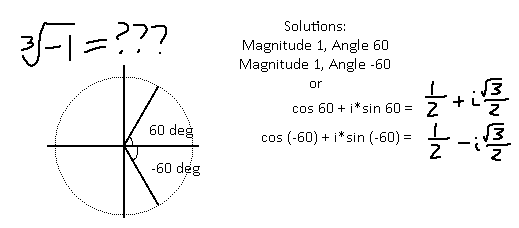Let's put this knowledge to the test: evaluate the sixth root of (-8), squared. The sixth root of (-8) is a question: What number, multiplied by itself 6 times, gives us -8? We are not dealing with unit values anymore, so this will be a little more tricky. I'm going to split this into two problems, so that we have a positive non-unit value by itself (8), and a potentially complex value by itself (-1).The term with the 8 in it is easier to handle, if you try to break it into 6 identical parts: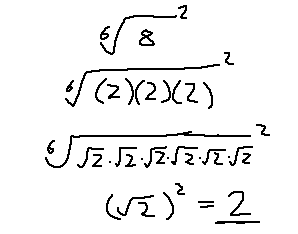The magnitude of our answer must be 2, then. Now all that's left is the (-1), which we can easily know solutions for, as we can stick with unit rotations. What rotation, added to itself 6 times, gives us a half-rotation? A twelve-rotation! So, this other term has a Magnitude of 1 and an Angle of 30 deg. Now that value has to be squared. Remember that squaring a value like this with a magnitude of 1 just means adding the angle by itself twice. This leaves us with a Magnitude of 1 and an Angle of 60 deg, or a sixth rotation around the unit circle. Don't forget that we can rotate the other direction, so our rotation can be 60 deg or -60 deg.

Here's the final steps and the resulting values in (a + bi) form: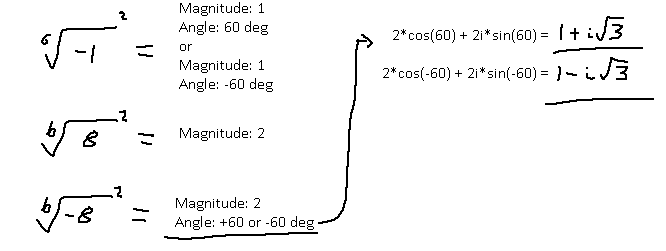An alternative route is to reduce the sixth root of a value squared so that it's just finding the cubed root of a value, and that should net you the same solutions.

Hopefully this makes finding complex values for roots much easier to understand.